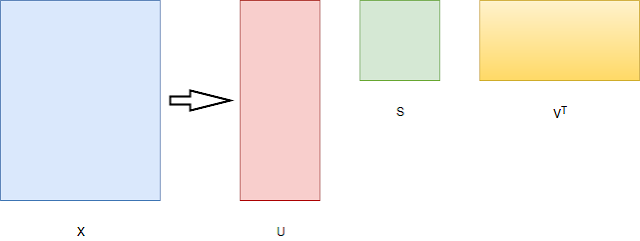# Word Embedding: Techniques

One of the most important aspects of any Natural Language Processing is the representation of the words. This throws up a unique problem of how to represent words in vector form. Words are ambiguous and can have multiple, complex relationships with other words. Additionally, the number of words in the vocabulary of any given language is in hundreds of thousands of magnitude.

One of the earliest attempts at word representation was made by WordNet, where each word was represented discretely and the relationships were established between words through hypernyms, synonyms etc. One of the main disadvantages of this setup was the amount of human intervention required to keep this corpus up-to-date. For a community that thrives on automation, this was just unacceptable. Additionally, this building of corpus was specific to a language. Building the corpus for any other language would require as much, if not more, effort and human intervention. Hence, this was not scalable.

Another discrete representation is one-hot representation (i.e creation of dummy variables). This is just a vector of size equal to the vocabulary size and each word is assigned an index. The one-hot vector of a word will be a vector filled with 0s with it's index bit set to 1. This representation also suffers from the same drawbacks as mentioned above. The vocabulary size of Google's corpus is about 13M. Hence each word would be required to be represented by a sparse vector of dimension 13M.

Another disadvantage of a one-hot representation is the underlying assumption that each word in the vocabulary is independent of each other, which is not the case. Since each word is represented by an arbitrary index, we cannot establish any implicit relationships between words.

In order to capture the semantics of any word and it's relationship to any other word, we need to look at the context in which the word appears. According to Richard Socher,
"You shall know a word by the company it keeps"
This gives us the idea that the representation of any word should be some function of the words surrounding the current word. One of the most intuitive ways of doing this is to use a co-occurrence matrix. This matrix just counts the number of times the current word is surrounded by another word.

There are two ways of taking the neighbours into account. One is to consider the document level frequency, where word frequencies are captured across documents. Second is to localize the context into neighbouring words (called windows). It is proven that the window based approach is better as it is capable of capturing both syntactic (Part-of-Speech) and semantic information.

The following is an example from Socher's slides, which better illustrates the co-occurrence matrix (for a window of size 1):One of the drawbacks of using co-occurrence matrix is that some words like 'is', 'the', 'at' etc, which have a high frequency of occurrence in a corpus can skew the importance of features towards themselves. Easiest way of overcoming this is to remove the stop-words and cap the maximum frequency of occurrence of any word.

Another improvement to the window method is to use a ramped window method where more importance is given to the words immediately next to the current word, than words which are much further away.Using this method, we can capture the semantics of the word up to some extent. But this method does not solve the problem of huge dimensionality of the word vector. It still remains the size of the vocabulary.

In order to reduce the dimension of the vector, we can leverage the age old linear algebraic method of Singular Value Decomposition (SVD).From Socher's slides,Here, $$S1, S2,...,Sn$$ are arranged according to the importance of the dimensions.

Although SVD is a simple way of reducing the dimensionality of the vector, it is computationally very expensive and it is difficult to add new words to the vocabulary (Since the matrix has to be decomposed every time the input matrix changes).

Hence, we use Word2Vec, a pseudo-neural approach of solving dimension reduction while preserving the semantic information of a co-occurrence matrix. There will be a separate post exploring Word2Vec in detail.

For more reference, refer Socher's slides at: Stanford: Word Vectors